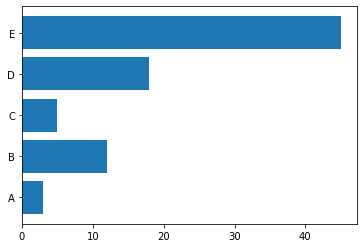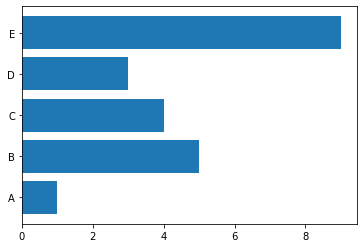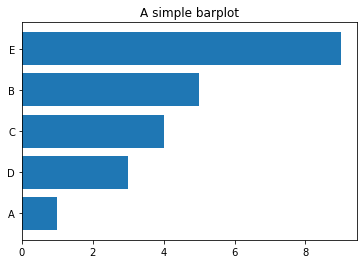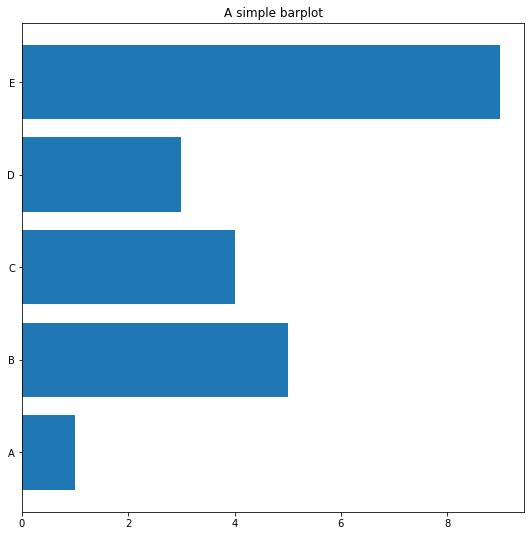# Plotting a Horizontal Barplot using Matplotlib

This post describes how to build a basic horizontal barplot using matplotlib.

## 📍 Most basic

Building a horizontal barplot with matplotlib follows pretty much the same process as a vertical barplot. The only difference is that the `barh()` function must be used instead of the `bar()` function. Here is a basic example.

``````# libraries
import matplotlib.pyplot as plt
import numpy as np

# create dataset
height = [3, 12, 5, 18, 45]
bars = ('A', 'B', 'C', 'D', 'E')
y_pos = np.arange(len(bars))

# Create horizontal bars
plt.barh(y_pos, height)

# Create names on the x-axis
plt.yticks(y_pos, bars)

# Show graphic
plt.show()``````## 🐼 From `Pandas` dataframe

The process is pretty much the same from a `Pandas` data frame

``````# import libraries
import pandas as pd
import matplotlib.pyplot as plt

# Create a data frame
df = pd.DataFrame ({
'Group':  ['A', 'B', 'C', 'D', 'E'],
'Value': [1,5,4,3,9]
})

# Create horizontal bars
plt.barh(y=df.Group, width=df.Value);

``````## ↕️ Control order

A bar chart is always more insightful when ordered. It allows to understand how items are ranked much quicker. You just have to sort the pandas dataframe upfront to sort the chart:

``````# import libraries
import pandas as pd
import matplotlib.pyplot as plt

# Create a data frame
df = pd.DataFrame ({
'Group':  ['A', 'B', 'C', 'D', 'E'],
'Value': [1,5,4,3,9]
})

# Sort the table
df = df.sort_values(by=['Value'])

# Create horizontal bars
plt.barh(y=df.Group, width=df.Value);

plt.title('A simple barplot');``````## ✨ Object-oriented API

Same graph as above using the matplotlib object `oriented API` instead of the `pyplot API`. See the matplotlib section for more about this.

``````# import libraries
import pandas as pd
import matplotlib.pyplot as plt

# Create a data frame
df = pd.DataFrame ({
'Group':  ['A', 'B', 'C', 'D', 'E'],
'Value': [1,5,4,3,9]
})

# Initialize a Figure and an Axes
fig, ax = plt.subplots()

# Fig size
fig.set_size_inches(9,9)

# Create horizontal bars
ax.barh(y=df.Group, width=df.Value);👋 This document is a work by Yan Holtz. Any feedback is highly encouraged. You can fill an issue on Github, drop me a message onTwitter, or send an email pasting `yan.holtz.data` with `gmail.com`.